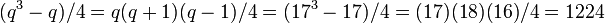# Projective special linear group:PSL(2,17)

Jump to: navigation, search
This article is about a particular group, i.e., a group unique upto isomorphism. View specific information (such as linear representation theory, subgroup structure) about this group
View a complete list of particular groups (this is a very huge list!)[SHOW MORE]

## Definition

This group is defined as the projective special linear group of degree two over field:F17.

## Arithmetic functions

Function Value Similar groups Explanation
order (number of elements, equivalently, cardinality or size of underlying set) 2448 groups with same order As$\! PSL(2,q), q = 17$,$q$ odd:$\! (q^3 - q)/2 = q(q+1)(q-1)/2 = (17^3 - 17)/2 = (17)(16)(18)/2 = 2448$. See element structure of projective special linear group of degree two.
exponent of a group 1224 groups with same order and exponent of a group | groups with same exponent of a group As$\! PSL(2,q), q = 17$,$q$ odd:$(q^3 - q)/4 = q(q+1)(q-1)/4 = (17^3-17)/4 = (17)(18)(16)/4 = 1224$. See element structure of projective special linear group of degree two.

### Arithmetic functions of a counting nature

Function Value Explanation
number of conjugacy classes 11 As$PSL(2,q), q = 17$,$q$ odd:$(q + 5)/2 = (17 + 5)/2 = 11$. See element structure of projective special linear group of degree two
number of conjugacy classes of subgroups 22
number of subgroups 2420

## Group properties

Property Satisfied? Explanation
abelian group No
nilpotent group No
solvable group No
simple group, simple non-abelian group Yes projective special linear group of degree two is simple (small exceptions exist)
minimal simple group Yes See classification of finite minimal simple groups

## GAP implementation

Description Functions used
PSL(2,17) PSL